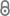# Time-Optimal Construction of Overlays

T. Götte, K. Hinnenthal, C. Scheideler, J. Werthmann, in: K. Censor-Hillel (Ed.), Proc. of the 40th ACM Symposium on Principles of Distributed Computing (PODC ’21), ACM, New York, n.d.Wicked_Fast_Overlay_Construction(1).pdf 590.88 KB
Conference Paper | Accepted | English
Author
Editor
Censor-Hillel, Keren
Abstract
We show how to construct an overlay network of constant degree and diameter $O(\log n)$ in time $O(\log n)$ starting from an arbitrary weakly connected graph. We assume a synchronous communication network in which nodes can send messages to nodes they know the identifier of and establish new connections by sending node identifiers. If the initial network's graph is weakly connected and has constant degree, then our algorithm constructs the desired topology with each node sending and receiving only $O(\log n)$ messages in each round in time $O(\log n)$, w.h.p., which beats the currently best $O(\log^{3/2} n)$ time algorithm of [Götte et al., SIROCCO'19]. Since the problem cannot be solved faster than by using pointer jumping for $O(\log n)$ rounds (which would even require each node to communicate $\Omega(n)$ bits), our algorithm is asymptotically optimal. We achieve this speedup by using short random walks to repeatedly establish random connections between the nodes that quickly reduce the conductance of the graph using an observation of [Kwok and Lau, APPROX'14]. Additionally, we show how our algorithm can be used to efficiently solve graph problems in \emph{hybrid networks} [Augustine et al., SODA'20]. Motivated by the idea that nodes possess two different modes of communication, we assume that communication of the \emph{initial} edges is unrestricted. In contrast, only polylogarithmically many messages can be communicated over edges that have been established throughout an algorithm's execution. For an (undirected) graph $G$ with arbitrary degree, we show how to compute connected components, a spanning tree, and biconnected components in time $O(\log n)$, w.h.p. Furthermore, we show how to compute an MIS in time $O(\log d + \log \log n)$, w.h.p., where $d$ is the initial degree of $G$.
Publishing Year
Proceedings Title
Proc. of the 40th ACM Symposium on Principles of Distributed Computing (PODC '21)
Conference
ACM Symposium on Principles of Distributed Computing (PODC)
Conference Location
Virtual
Conference Date
2021-07-26 – 2021-07-30
LibreCat-ID

### Cite this

Götte T, Hinnenthal K, Scheideler C, Werthmann J. Time-Optimal Construction of Overlays. In: Censor-Hillel K, ed. Proc. of the 40th ACM Symposium on Principles of Distributed Computing (PODC ’21). New York: ACM. doi:10.1145/3465084.3467932
Götte, T., Hinnenthal, K., Scheideler, C., & Werthmann, J. (n.d.). Time-Optimal Construction of Overlays. In K. Censor-Hillel (Ed.), Proc. of the 40th ACM Symposium on Principles of Distributed Computing (PODC ’21). New York: ACM. https://doi.org/10.1145/3465084.3467932
@inproceedings{Götte_Hinnenthal_Scheideler_Werthmann, place={New York}, title={Time-Optimal Construction of Overlays}, DOI={10.1145/3465084.3467932}, booktitle={Proc. of the 40th ACM Symposium on Principles of Distributed Computing (PODC ’21)}, publisher={ACM}, author={Götte, Thorsten and Hinnenthal, Kristian and Scheideler, Christian and Werthmann, Julian}, editor={Censor-Hillel, KerenEditor} }
Götte, Thorsten, Kristian Hinnenthal, Christian Scheideler, and Julian Werthmann. “Time-Optimal Construction of Overlays.” In Proc. of the 40th ACM Symposium on Principles of Distributed Computing (PODC ’21), edited by Keren Censor-Hillel. New York: ACM, n.d. https://doi.org/10.1145/3465084.3467932.
T. Götte, K. Hinnenthal, C. Scheideler, and J. Werthmann, “Time-Optimal Construction of Overlays,” in Proc. of the 40th ACM Symposium on Principles of Distributed Computing (PODC ’21), Virtual.
Götte, Thorsten, et al. “Time-Optimal Construction of Overlays.” Proc. of the 40th ACM Symposium on Principles of Distributed Computing (PODC ’21), edited by Keren Censor-Hillel, ACM, doi:10.1145/3465084.3467932.
Main File(s)
File Name
Wicked_Fast_Overlay_Construction(1).pdf 590.88 KB
Access LevelClosed Access